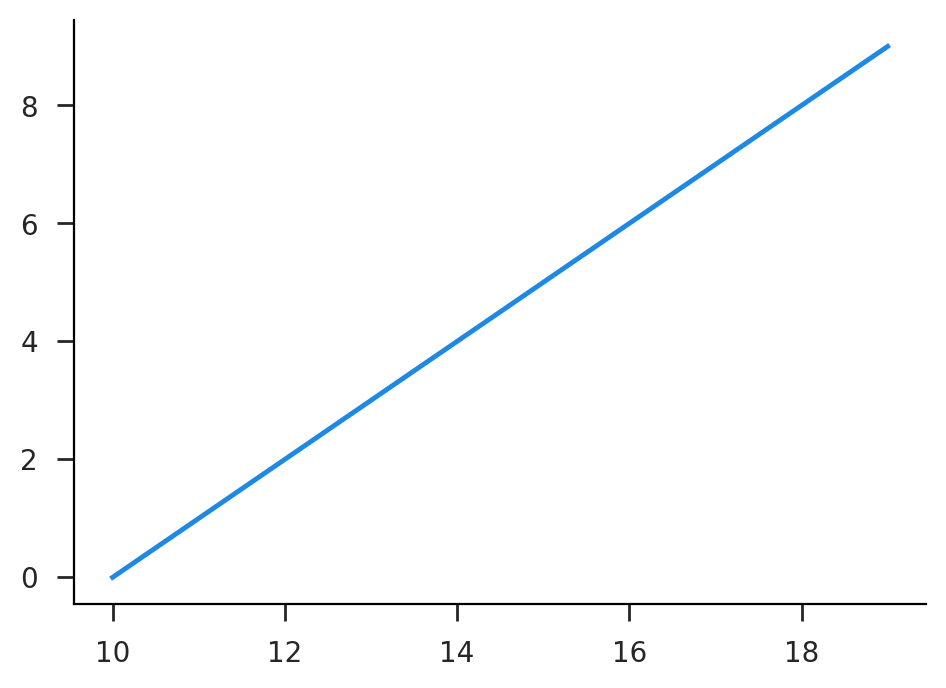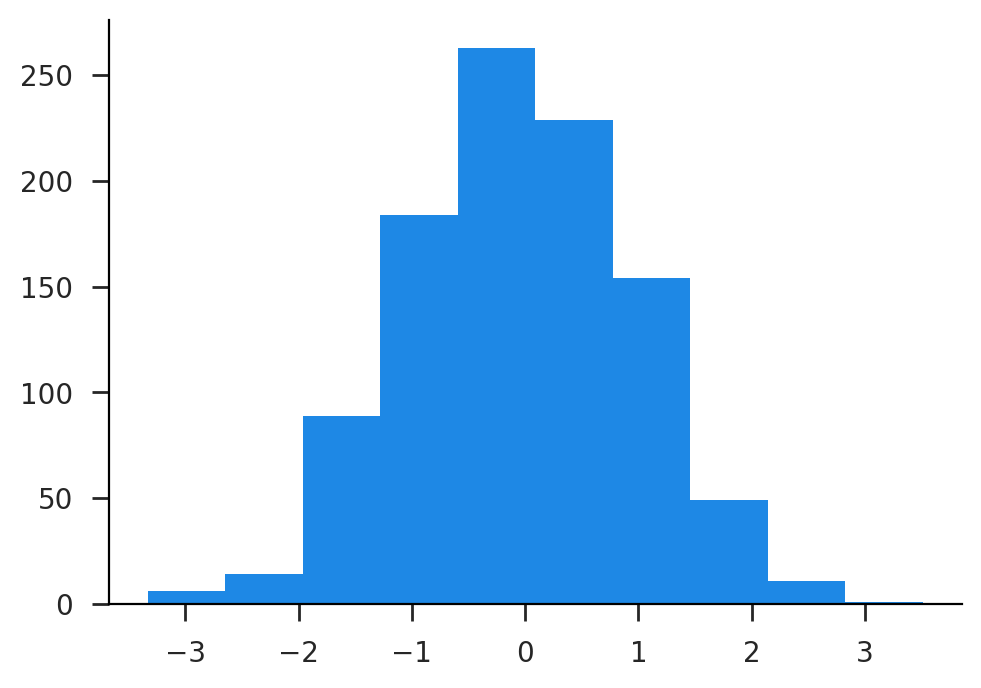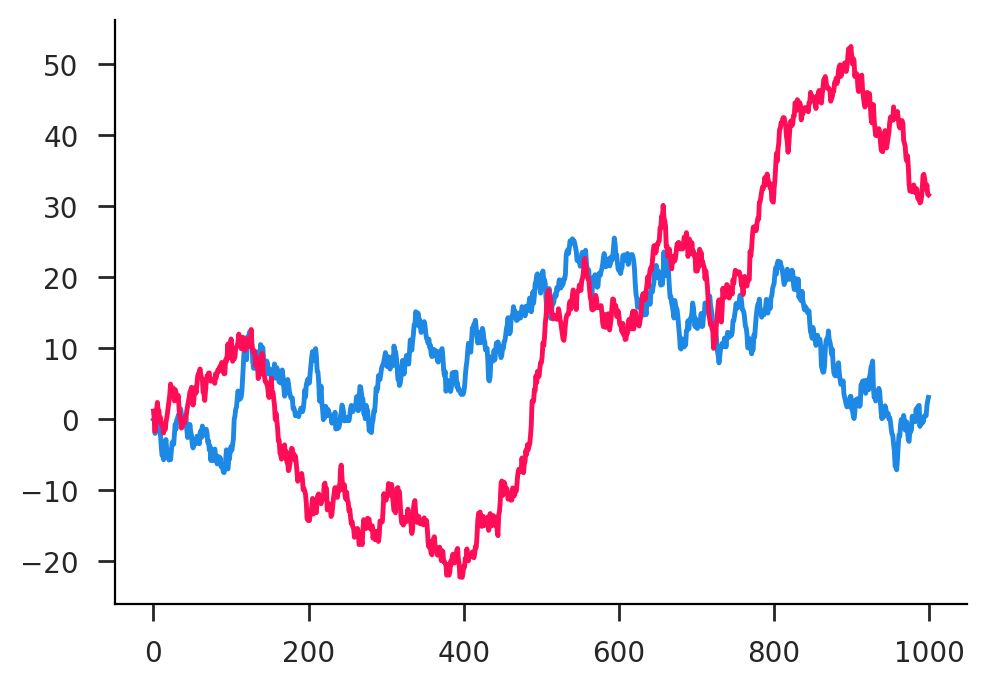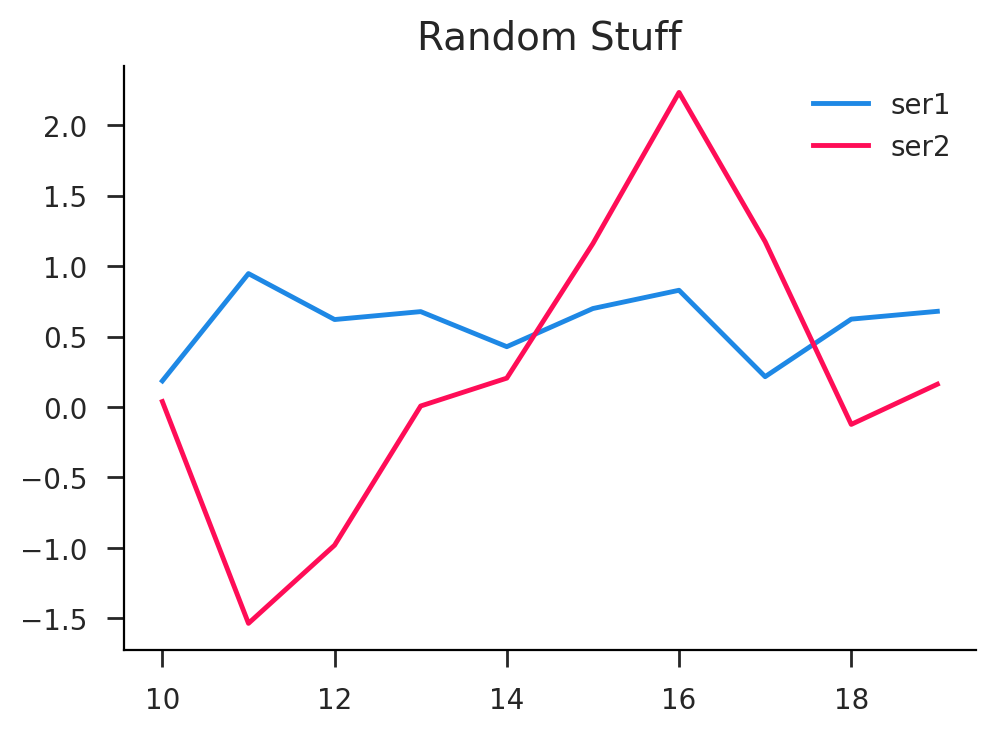# Lecture 0: Python & Ecosystem¶

Yes, we're zero indexing!

# Introduction¶

### Git¶

Git is a version control system that runs on your computer. You can commit changes to the repository and it will save a history of these commits, allowing you to reference them or go back to them at any time. GitHub is a hosting service that essentially backs up your Git repositories (when you push to them or pull from them) so that you can acccess them from anywhere and there is a reduced chance of data loss. You can also check out other people's GitHub repository by clone-ing them.

### Python¶

There are basically two ways to intall Python. One is the "native" way using whatever facilities exist with your operating system (for OSX this would be homebrew), and then using pip to install packages. The other way, which is generally more user-friendly and OSX/Windows friendly is to use the Anaconda distribtuion and conda to install packages.

The most bare-bones way to run Python is to just execute python in the terminal. Depending on your setup, this might actually run Python 2, which is quite old at this point, so it's often safer to run python3 explicitly. Anyway, you'll almost never need to do this. At the very least you'll want to run in a more user friendly environment like IPython by executing ipython3, which is sort of a wrapper around python3.

But even then, most of you will prefer to use Jupyterlab. This is a web-based graphical interface that is primary centered on "notebooks", which is what you're looking at now basically. It's just a series of cell (code or markdown) that when run produce some kind of output. Notebooks are a way to store the code you've written and the resulting output in one place.

The other place where Python code might "live" is in Python files, which are just text files with the extension .py. If you have a file named model.py, you can run its contents directly with python3 model.py. If you're in an IPython terminal and you want to run its contents interactively, you can run run -i model.py. Finally, you can use it as a module. In this case you can run the Python command import model and there will be a new variable model that contains any variables defined therein. So if you'd defined abc = 5 in model.py then model.abc will be 5.

### Jupyter¶

Nowadays, you can do almost everything in Jupyterlab. That can be useful, especially if you're working on a remote machine like the Pitt cluster. However, I must emphasize that you shouldn't be doing everything in notebooks. For suffiently complex code, you'll want to put some portions of it proper Python modules (.py files, basically) and import them for usage in a notebook. Thus your notebook will contain mostly high level commands and their resulting outputs.

You can create a new notebook (.ipynb file) by clicking on the blue "+" on the left and chooseing a Python version (something like 3.9 or higher is recommended). You can also create other file types like Python (.py) or markdown (.md) or open a system terminal. Finally, you can edit any of these files by double clicking on them in the filesystem pane on the left.

### Tips¶

You'll want to stick mostly to the keyboard. To run a cell, press Shift+Enter. To run a bunch of cells in a row, just hold down Shift and keep pressing Enter. To enter edit mode on the selected cell, press Enter. To exit edit mode on a cell, just press Esc. To interrupt ongoing execution, press i twice. To completely restart a notebook press0 twice. Create new cells above or below with a and b.

You can make a cell into a markdown cell by pressing m. Press y to turn it back into a code cell. In markdown mode, you can make headings with one or more #s, amongst other markdown features such as pairs of ** for bold text. You can also do inline $\LaTeX$-style math with pairs of $, as in$x^2$, or display style math with pairs of $$, as in$$ \int_{-\infty}^{\infty} \exp(-x^2) dx = \sqrt{\pi}$\$

# Python Basics¶

### Tuples¶

There are a small number of core data types that are quite powerful. First there's the tuple which is basically a list of objects

In :
a = (1, 2, 'abc')
a

Out:
(1, 2, 'abc')

When the grouping is not ambiguous, you can omit the parenthases

In :
a = 1, 2, 'abc'
a

Out:
(1, 2, 'abc')

In the other direction, you can unpack tuples and assign their members to separate variables

In :
b, c, d = a
c

Out:
2

You can select subsets of a tuple by slicing them

In :
a[1:]

Out:
(2, 'abc')

### Lists¶

Tuples aren't super flexible. Once you've created them, you can't resassign their elements, though you can append new ones to the end. For more interactive use cases, you'll want to use a list. They look and act a lot like tuples, but you can modify them

In :
a = [1, 2, 'abc']
print(a)
a.append(5)
print(a)
a = 10
print(a)

[1, 2, 'abc']
[1, 2, 'abc', 5]
[1, 10, 'abc', 5]


There are a couple of fancy operations you can do with lists that use overloaded algebraic operators

In :
a = [1, 2, 3]
b = [4, *a, 10]
print(b)
c = a + b
print(c)
d = 3*a
print(d)

[4, 1, 2, 3, 10]
[1, 2, 3, 4, 1, 2, 3, 10]
[1, 2, 3, 1, 2, 3, 1, 2, 3]


Here you can see that using * in front of a list variable acts as if you had typed out the contents.

### Dictionaries¶

I would say that the dict is the quintessential type on Python. They are extremely useful and many things use them. I dict is just a mapping between different objects, from keys to values. The values can be of any type, which they keys are restricted to being "hashable", which includes things like numbers, strings, and tuples (but not lists).

In :
d = {1: 2, 'abc': 10, 12: 'foo'}
d

Out:
{1: 2, 'abc': 10, 12: 'foo'}

You can access the elements of dictionaries with square brackets

In :
d

Out:
'foo'

You can combine dicts as we saw with lists but using ** instead.

In :
e = {**d, 15: 1}
e

Out:
{1: 2, 'abc': 10, 12: 'foo', 15: 1}

### For Loops¶

You can loop over iterables like tuples, lists, and other things using for loops.

In :
for i in [1, 2, 3, 4]:
print(2*i)

2
4
6
8


There's also something known as a list comprehension that lets you do this in more compact form

In :
a = range(5) # generates a list from 0 to 4, inclusive
b = [2*i for i in a]
print(b)

[0, 2, 4, 6, 8]


We can also do comprehensions on dictionaries

In :
a = [1, 2, 3, 4]
{i: 2*i for i in a}

Out:
{1: 2, 2: 4, 3: 6, 4: 8}

### Functions¶

Functions are similar to other programming languages. But they can also be assigned and passed around like variables

In :
def add(x, y):
return x + y

Out:
6

For smaller functions, you can also use the lambda function notation

In :
add = lambda x, y: x + y

Out:
6

### Zip¶

You can combine multiple iterables together using zip. This turns out to pretty useful

In :
a = [1, 2, 3, 4, 5]
b = [10, 11, 12, 13, 14]
zip(a, b)

Out:
<zip at 0x7f1d091f2500>

Ok, that seems less useful. It turns out zip returns an iterator object instead of the real thing. There are good efficiency reasons for this, but to get it to give you the real values, you need to do

In :
list(zip(a, b))

Out:
[(1, 10), (2, 11), (3, 12), (4, 13), (5, 14)]

### Modules¶

There are quite a few built in modules that have useful functions. There are also many third-party modules that we'll use extensively.

In :
import re # regular expressions
re.sub(r'\d', 'x', 'My phone number is 123-4567')

Out:
'My phone number is xxx-xxxx'

Here's an example using itertools.chain which is often useful for chaining iterators together. In addition to itertools, other all-star built-in modules include operator, functools, and operator.

In :
from itertools import chain
a = [range(i) for i in range(5)]
print(a)
b = chain(*a)
list(b)

[range(0, 0), range(0, 1), range(0, 2), range(0, 3), range(0, 4)]

Out:
[0, 0, 1, 0, 1, 2, 0, 1, 2, 3]

# Libraries¶

### Numerics with numpy¶

In :
# it's pronounced num-pie :)
import numpy as np


The central object in numpy is an N-dimensional array type np.ndarray. Lots of stuff here is going to be similar to matlab arrays.

In :
np.ones(10)

Out:
array([1., 1., 1., 1., 1., 1., 1., 1., 1., 1.])

You can create an array from a list with np.array, but the inputs should be numerical

In :
np.array([1, 2, 3, 4])

Out:
array([1, 2, 3, 4])

Note that when generating ranges, the left limit is inclusive while the right limit is non-inclusive

In :
a = np.arange(10)
print(a)

[0 1 2 3 4 5 6 7 8 9]


There are a bunch of different ways to slice arrays, much like lists but more powerful.

In :
a = np.arange(10)
print(a)
print(a) # zero indexed!
print(a[3:]) # no 'end' needed
print(a[:-1]) # negatives index from end
print(a[3:5]) # second index is non-inclusive
print(a[[4,1,9,2]]) # index with a list

[0 1 2 3 4 5 6 7 8 9]
0
[3 4 5 6 7 8 9]
[0 1 2 3 4 5 6 7 8]
[3 4]
[4 1 9 2]


We can "broadcast" new dimensions at will. Here we make a column vector. Note that the row dimension is the first index (row-major)

In :
a[:, None]

Out:
array([,
,
,
,
,
,
,
,
,
])

Here's same thing but for a row vector

In :
a[None, :]

Out:
array([[0, 1, 2, 3, 4, 5, 6, 7, 8, 9]])

We can construct complex matrices using indexing and broadcasting. Do this instead of repmat!

In :
a[:, None] + a[None, :]

Out:
array([[ 0,  1,  2,  3,  4,  5,  6,  7,  8,  9],
[ 1,  2,  3,  4,  5,  6,  7,  8,  9, 10],
[ 2,  3,  4,  5,  6,  7,  8,  9, 10, 11],
[ 3,  4,  5,  6,  7,  8,  9, 10, 11, 12],
[ 4,  5,  6,  7,  8,  9, 10, 11, 12, 13],
[ 5,  6,  7,  8,  9, 10, 11, 12, 13, 14],
[ 6,  7,  8,  9, 10, 11, 12, 13, 14, 15],
[ 7,  8,  9, 10, 11, 12, 13, 14, 15, 16],
[ 8,  9, 10, 11, 12, 13, 14, 15, 16, 17],
[ 9, 10, 11, 12, 13, 14, 15, 16, 17, 18]])

Multiplication is element-wise by default (like .* in matlab)

In :
np.arange(10) * np.arange(10)

Out:
array([ 0,  1,  4,  9, 16, 25, 36, 49, 64, 81])

In :
np.arange(10, 20)[None, :]*np.arange(5,15)[:, None]

Out:
array([[ 50,  55,  60,  65,  70,  75,  80,  85,  90,  95],
[ 60,  66,  72,  78,  84,  90,  96, 102, 108, 114],
[ 70,  77,  84,  91,  98, 105, 112, 119, 126, 133],
[ 80,  88,  96, 104, 112, 120, 128, 136, 144, 152],
[ 90,  99, 108, 117, 126, 135, 144, 153, 162, 171],
[100, 110, 120, 130, 140, 150, 160, 170, 180, 190],
[110, 121, 132, 143, 154, 165, 176, 187, 198, 209],
[120, 132, 144, 156, 168, 180, 192, 204, 216, 228],
[130, 143, 156, 169, 182, 195, 208, 221, 234, 247],
[140, 154, 168, 182, 196, 210, 224, 238, 252, 266]])

You can always get shape/size information about an array.

In :
a = np.ones((3, 5))
print(a.shape, a.size)
a

(3, 5) 15

Out:
array([[1., 1., 1., 1., 1.],
[1., 1., 1., 1., 1.],
[1., 1., 1., 1., 1.]])

Reshaping is a whole thing.

In :
a = np.ones((4, 5))
print(a.reshape((10, 2)).shape)
print(a.T.shape)
print(a.flatten().shape)

(10, 2)
(5, 4)
(20,)


There is basic linear algebra in numpy, but you'll want to see scipy for more advanced operations and for statistical distributions.

In :
m = np.random.rand(5, 5)
mi = np.linalg.inv(m)
print(mi)
print((np.dot(m, mi)-np.eye(5)).max())

[[ 0.17941876  1.8425588   3.5254179  -2.64382421 -0.71398209]
[-1.69050323 -2.30513917 -3.17945327  3.36489942  2.21657208]
[ 0.18185687  1.30491758  0.26778018 -1.29656829  0.67987751]
[ 4.4767183   2.05611406  5.83024995 -5.07426302 -3.99665051]
[-2.3655925  -1.98204086 -4.80832243  5.23262069  1.3236275 ]]
9.211347777390836e-16


But numpy has many different routines for random number generation.

In :
np.random.randint(5, size=10)

Out:
array([0, 1, 3, 2, 3, 4, 4, 4, 4, 0])

### Plotting with matplotlib¶

First I'm going to do some non-required stuff to configure graph appearance to my liking

In :
import matplotlib as mpl
mpl.style.use('./config/clean.mplstyle') # this loads my personal plotting settings
%config InlineBackend.figure_format = 'retina' # if you have an HD display


For most use cases, this is the only import you need. Note that it is a little non-standard.

In :
import matplotlib.pyplot as plt


First let's do a simple line plot example. You'll usually be passing numpy arrays to matplotlib, but it also accepts lists.

In :
plt.plot(np.arange(10, 20), np.arange(10));Another useful plot is the histogram for a given array.

In :
plt.hist(np.random.randn(1000));Passing a 2d array will treat each column as a separate series.

In :
plt.plot(np.cumsum(np.random.randn(1000, 2), axis=0));### Data with pandas¶

In :
import pandas as pd


A Series is a 1-D array with an attached index, which defaults to range(n).

In :
s = pd.Series(np.random.rand(10), index=np.arange(10, 20))
s

Out:
10    0.185459
11    0.947054
12    0.620941
13    0.677210
14    0.428261
15    0.699120
16    0.828754
17    0.215353
18    0.623805
19    0.679220
dtype: float64

Let's look at the underlying data

In :
print(s.index)
print(s.values)

Int64Index([10, 11, 12, 13, 14, 15, 16, 17, 18, 19], dtype='int64')
[0.18545867 0.94705393 0.62094138 0.67720985 0.4282612  0.69912019
0.82875414 0.21535327 0.62380505 0.67921995]


Or get a quick summary of a numeric series

In :
s.describe()

Out:
count    10.000000
mean      0.590518
std       0.245908
min       0.185459
25%       0.476431
50%       0.650507
75%       0.694145
max       0.947054
dtype: float64

A DataFrame is like a dictionary of Series with a common index

In :
df = pd.DataFrame({'ser1': s, 'ser2': np.random.randn(10)})

Out:
ser1 ser2
10 0.185459 0.038782
11 0.947054 -1.535639
12 0.620941 -0.980943
13 0.677210 0.007174
14 0.428261 0.205036

We can get summary stats for each column.

In :
df.describe()

Out:
ser1 ser2
count 10.000000 10.000000
mean 0.590518 0.234001
std 0.245908 1.083645
min 0.185459 -1.535639
25% 0.476431 -0.090772
50% 0.650507 0.100324
75% 0.694145 0.921442
max 0.947054 2.233067

This makes plotting much more convenient and powerful.

In :
df.plot(title='Random Stuff');Accessing individual columns yields a Series

In :
df['ser1']

Out:
10    0.185459
11    0.947054
12    0.620941
13    0.677210
14    0.428261
15    0.699120
16    0.828754
17    0.215353
18    0.623805
19    0.679220
Name: ser1, dtype: float64

We can perform vector operations on these

In :
df['ser1'] > 0.5

Out:
10    False
11     True
12     True
13     True
14    False
15     True
16     True
17    False
18     True
19     True
Name: ser1, dtype: bool

We can select particular rows in this way.

In :
df1 = df[(df['ser1']>0.5) & (df['ser2']<1.0)]
df1

Out:
ser1 ser2
11 0.947054 -1.535639
12 0.620941 -0.980943
13 0.677210 0.007174
18 0.623805 -0.123421
19 0.679220 0.161867

### Regression with statsmodels¶

This import is also non-standard. We're going to use the formula based API.

In :
import statsmodels.formula.api as smf


Gererate some random data with a known causal structure.

In :
N = 100
x = np.random.randn(N)
y = 3*np.random.randn(N)
z = 1 + 2*x + 3*y + 4*x*y + np.random.randn(N)
df0 = pd.DataFrame({'x': x, 'y': y, 'z': z})


Run an OLS regression with a properly specified model.

In :
ret = smf.ols('z ~ 1 + x + y + x:y', data=df0).fit()
ret.summary()

Out:
Dep. Variable: R-squared: z 0.995 OLS 0.995 Least Squares 6051. Wed, 18 Oct 2023 3.20e-109 01:17:27 -129.82 100 267.6 96 278.1 3 nonrobust
coef std err t P>|t| [0.025 0.975] 0.9803 0.091 10.820 0.000 0.800 1.160 1.9855 0.081 24.553 0.000 1.825 2.146 3.0253 0.036 83.635 0.000 2.954 3.097 3.9928 0.033 120.989 0.000 3.927 4.058
 Omnibus: Durbin-Watson: 1.599 2.149 0.45 1.284 -0.063 0.526 2.459 3.07

Notes:
 Standard Errors assume that the covariance matrix of the errors is correctly specified.

You can access the parameters and standard errors directly as a series and dataframe

In :
print(ret.params)
print(ret.cov_params())

Intercept    0.980283
x            1.985538
y            3.025330
x:y          3.992819
dtype: float64
Intercept         x         y       x:y
Intercept   0.008208  0.000008 -0.000096 -0.000162
x           0.000008  0.006539 -0.000104  0.000110
y          -0.000096 -0.000104  0.001308  0.000300
x:y        -0.000162  0.000110  0.000300  0.001089

In [ ]: